Question

The sum of three odd consecutive numbers is fifty-one. What are the numbers?

1.15,17,19

Step-by-step explanation:

Make an equation :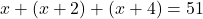Solve the equation: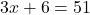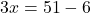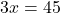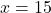Since x=15 then the second number is 17 and then 19

Hope this helps!

Stay Safe